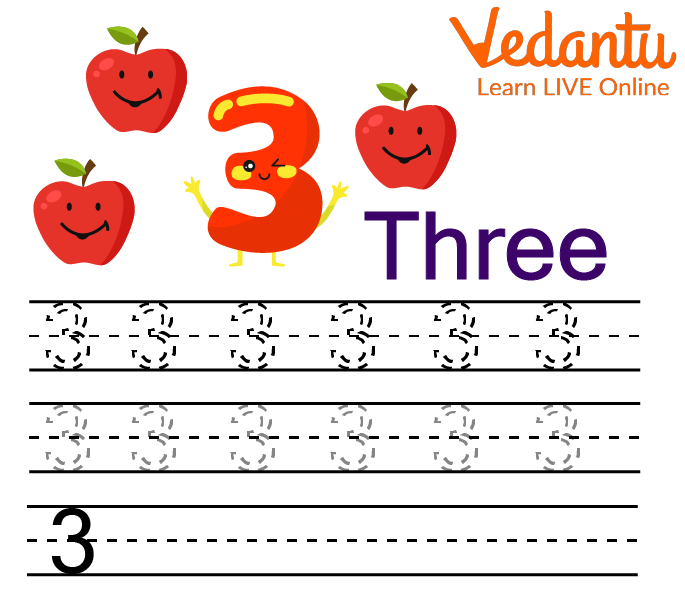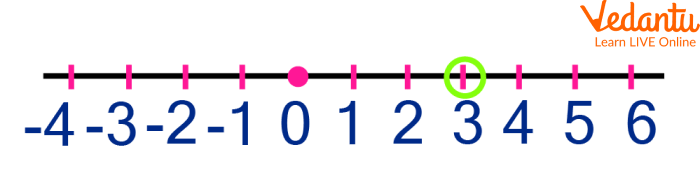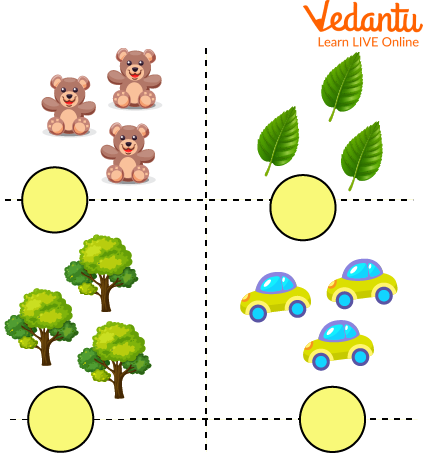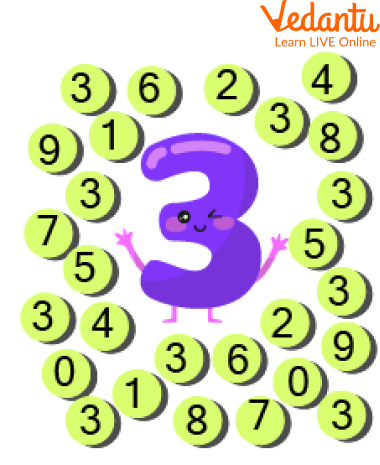Courses
Courses for Kids
Free study material
Free LIVE classes
More

# Identify Number 3## Overview of the Number 3

Are you stabbed worrying about how to teach your children to identify number 3? If yes, then you have come across the right platform. This article will aid you in teaching 3 to the children by using number 3 activities. Here, we have discussed the number 3, its different writing forms, its representation on the number line, and many more. This writing helps in making the learning process simple and interesting. Now, are you ready to start memorizing this interesting topic?

## What is the Number 3?

A number, numeral, and digit, 3 is defined as the natural number that comes after 2 and before 4. It is the smallest possible prime number which is an odd number. Divisors of 3 are 1, and 3 itself. In many societies and cultures, the number 3 is considered religious and it also possesses certain cultural implications.

## Writing Number 3 in different Formats

The number 3 can be written in different forms such as:

• In Number Names

In terms of number names, 3 is written as three

• In Ordinal Form

## In terms of ordinal form, the number 3 is written as third

In roman numeral form, the number 3 is depicted by writing it as lll

It can not be expressed in terms of the square of a number

## Number 3 with Objects

Number 3 is the counting number, which is represented by using the objects, in the below-given images. The use of graphics helps the parents and teachers to teach number 3 to the children easily.Showing number 3 with objects

## How to write Number 3?

To learn the number 3, one should practice writing it again and again by counting. Some worksheets are given below that are based on the concept of the dotted phenomenon which aids the children in learning how to write the number 3 easily.How to write Number 3

## Solved Examples

Q1. Can the number 9 be expressed in terms of 3?

Ans. Yes, the number 9 can be expressed in terms of 3 because it is the square root of the number 9 I.e. multiplying 3 with 3 , we get 9.

Thus, the number 3 is the square root of the number 9.

Q2. How to represent the number 3 on the number line?

Ans. To represent the number 3 on the number line, follow the given steps:

1. Draw a straight line of any length using the scale

2. Represent the integers on the number line

3. Mark the point where you get the number 3

Thus, the number 3 is represented on the number line.Number 3 on number line

## Practice Problems

Till now, the children would gain a clear picture of the number 3 in their mind which would help them to identify it easily. Some number 3 worksheets for nursery are given below along with graphics that help the tutors in evaluating how much the kids have gained and whether they can identify the number 3 or not.

Q1. Write the number of objects in the circle shown in each box:Number 3 worksheet for nursery, based on count and write

Q2. How many 3’s are there in the below image?Number 3 worksheet for nursery, based on the concept of circling

## Summary

To wrap up here with the topic of learning the number 3. It serves as the fundamental block in memorizing mathematics. It is useful not only in mathematics but also in real life. This article covers every topic related to number 3 by using number 3 activities and graphics so that children would easily grab the topic. The language used in the above writing is kept simple and fascinating to make the understanding of the concepts deeper. I Hope you find the article useful and enjoy reading it.

Last updated date: 23rd Sep 2023
Total views: 77.7k
Views today: 0.77k

## FAQs on Identify Number 3

1. What are whole numbers?

Whole numbers are defined as a combined set of counting numbers and zero. These always start from 0 but do not have any ending point. The English letter, W is used to represent whole numbers.

W = 0, 1, 2, 3, 4, 5, …………

2. Define a real number line.

A real number line is defined as the visual representation of numbers on a straight or horizontal line. Both ends of the number line point to infinity. One can represent any set of numbers on the number line be it natural numbers, whole numbers, integers, and rational and irrational numbers. Simply, it can be said that the number line is used to represent the set of real numbers. Sometimes, a real number line is also called the real line or simply a  number line.

3. Is 0 included in the set of natural numbers?

No, zero is not included in the set of natural numbers. If the natural numbers are written along with zero, these will form a new set of numbers, called whole numbers, which are represented by the English letter W.